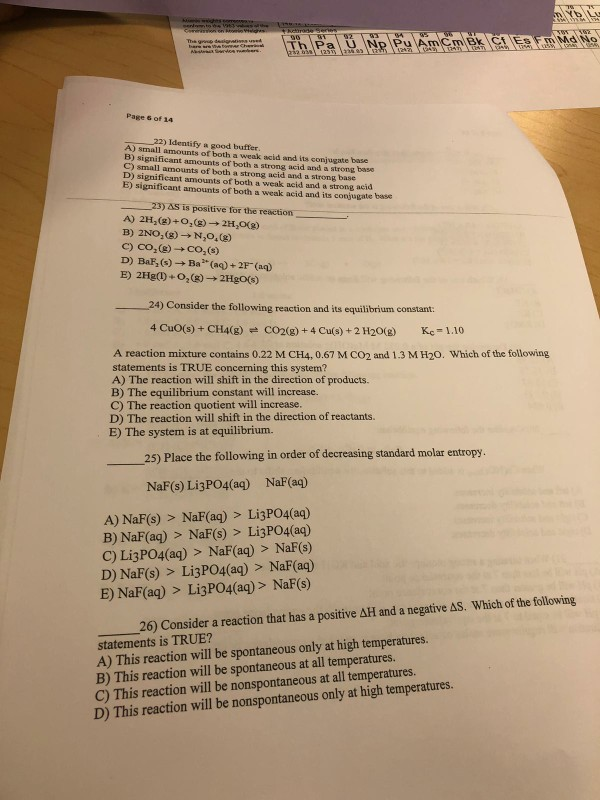# pls do all Page 6 of 14 22) Identify a good buffer. ) small amounts of both a weak acid and its conjugate base B) significant amounts of both a strong acid and a strong bso C) small amounts of bot...pls do all

Page 6 of 14 22) Identify a good buffer. ) small amounts of both a weak acid and its conjugate base B) significant amounts of both a strong acid and a strong bso C) small amounts of both a strong acid and a strong base D) significant amounts of both a weak acid and a strong acid E) significant amounts of both a weak acid and its conjugate base 23) AS is positive for the reaction A) 2H2(g) +02(g)→2H2O(g) C) CO2(g) . D) BaF,(s)- Co,(s) Ba(a)+2F(a) 24) Consider the following reaction and its equilibrium constant: 4 CuO(s) + CH4(g)-CO2(g) + 4 Cu(s) +2 H2O(g) Kc = 1.10 A reaction mixture contains 0.22 M CH4, 0.67 M CO2 and 1.3 M H20. Which of the following statements is TRUE concerning this system? A) The reaction will shift in the direction of products. B) The equilibrium constant will increase. C) The reaction quotient will increase. D) The reaction will shift in the direction of reactants. E) The system is at equilibrium. 25) Place the following in order of decreasing standard molar entropy NaF(s) LigPO4(aq) NaF(aq) A) NaF(s) > NaF(aq) > Li3PO4(aq) B) NaF(aq) > NaF(s) > Li3PO4(aq) C) Li3PO4(aq) > NaF(aq) > NaF(s) D) NaF(s) > Li3PO4(aq) > NaF(aq) E) NaF(aq) > Li3PO4(aq)> NaF(s) 26) Consider a reaction that has a positive AH and a negative AS. Which of the following statements is TRUE? A) This reaction will be spontaneous only at high temperatures. B) This reaction will be spontaneous at all temperatures. C) This reaction will be nonspontaneous at all temperatures. D) This reaction will be nonspontaneous only at high temperatures.

22) Answer is E). Strong acid and base don't act as good buffer.

23) Answer is D). Because product is aqueous which has high entropy than solid reactant.

Reaction quotient,Q = [CO2][H2O]2/[CH4] = 0.67×(1.3)2/0.22

Q = 5.147

Since Q>K hence reaction go backward

25) Answer is C). Solid has low entropy and higher the ions higher entropy.

26) Answer is C). As ∆G = ∆H-T∆S so, ∆G is positive hence reaction is non-spontaneous at all temperature.

##### Add Answer of: pls do all Page 6 of 14 22) Identify a good buffer. ) small amounts of both a weak acid and its conjugate base B) significant amounts of both a strong acid and a strong bso C) small amounts of bot...
More Homework Help Questions Additional questions in this topic.

• #### pKa Conjugate base Acid formula Conjugate base name Acid name F3CCOOH 0.20 F3ccoo trifluoroacetic acid trifluoroacetate...

Need Online Homework Help?